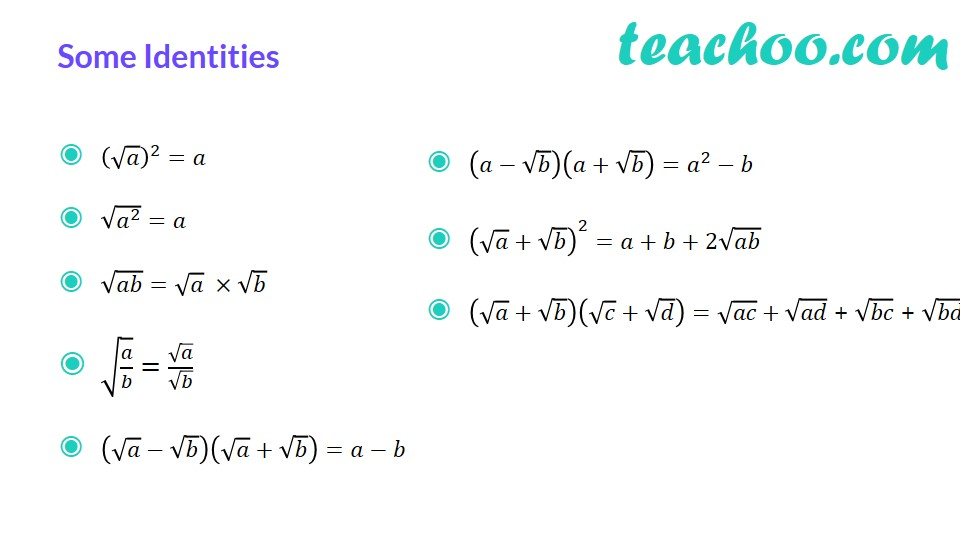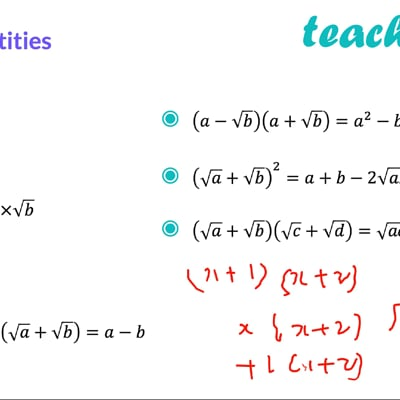Rationalising

Chapter 1 Class 9 Number Systems (Term 1)
Concept wiseThis video is only available for Teachoo black users

We do not leave an irrational number in the denominator.

So, we rationalise the denominator.  Let us look at some examples

### Transcript

Some Identities (√𝑎)^2=𝑎 √(𝑎^2 )=𝑎 √𝑎𝑏=√𝑎 ×√𝑏 √(𝑎/𝑏)=√𝑎/√𝑏 (√𝑎−√𝑏)(√𝑎+√𝑏)=𝑎−𝑏 (𝑎−√𝑏)(𝑎+√𝑏)=𝑎^2−𝑏 (√𝑎+√𝑏)^2=𝑎+𝑏+2√𝑎𝑏 (√𝑎+√𝑏)(√𝑐+√𝑑)=√𝑎𝑐+√𝑎𝑑 + √𝑏𝑐 + √𝑏𝑑